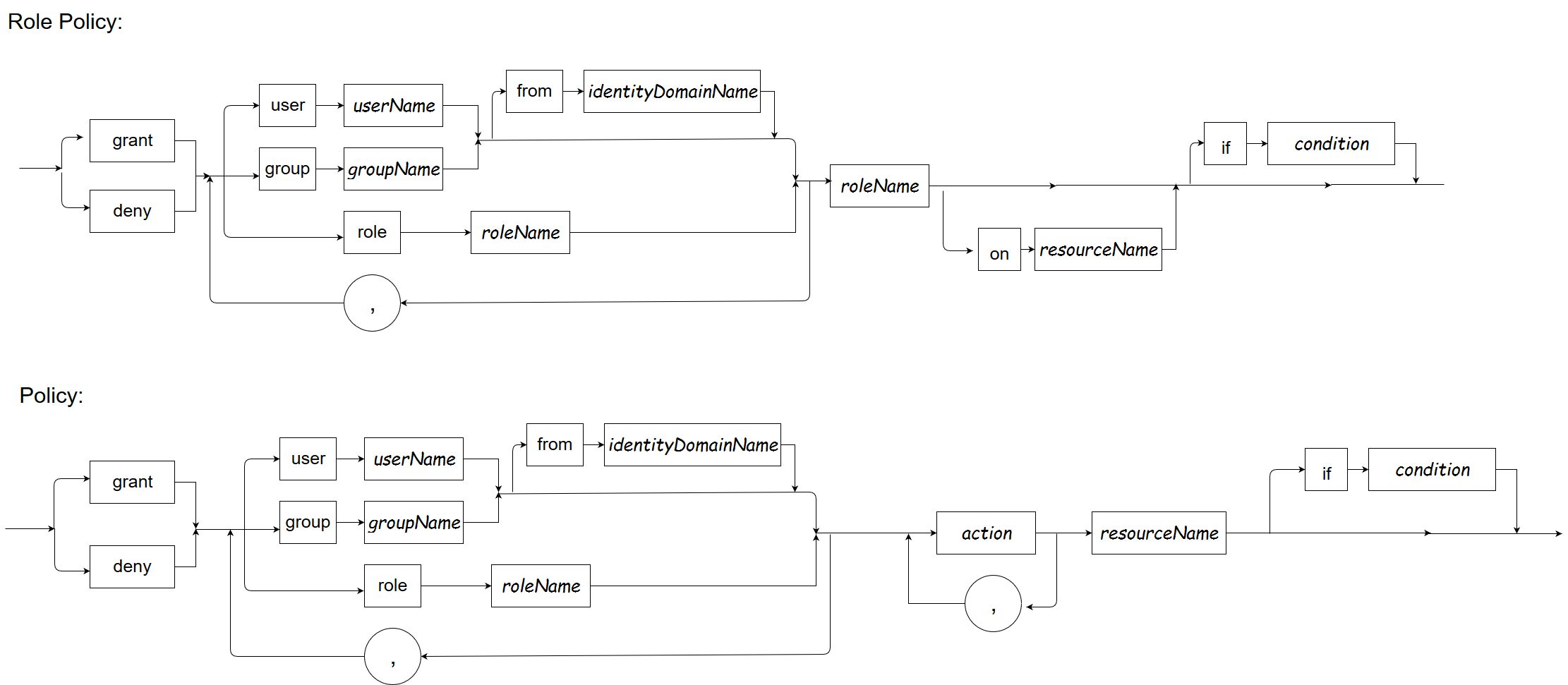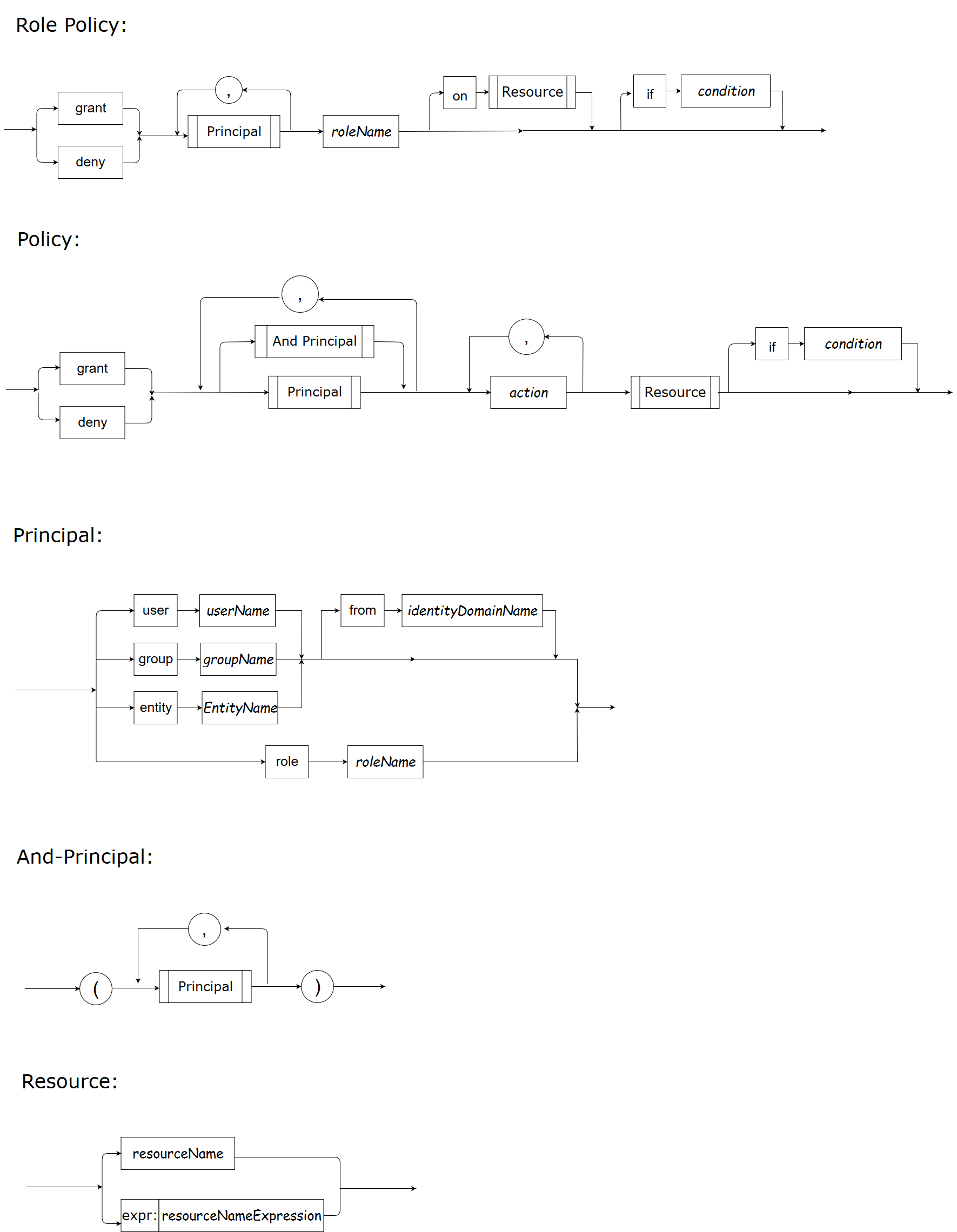SPDL - Security Policy Definition Language

Basics of SPDL

SyntaxKeywords

The reserved keywords in SPDL are as follows. You cannot use any of these keywords as user name, group name, action, resource, attribute name, and so on.

• role
• user
• group
• entity
• grant
• deny
• if
• in
• on
• from

The keywords are all case-insensitive, which means that you cannot use one of “role”, “ROLE”, “Role”, “rOLe”, etc as user name, group name, action, resource, attribute name, and so on.

Naming Convention

User Name: a user name consists of letters, decimal digits, punctuation marks except for comma  i.e. [\p{L}\p{Nd}[\p{Punct}&&[^,]]]+

Group Name: a group name consists of letters, decimal digits, punctuation marks except for comma i.e. [\p{L}\p{Nd}[\p{Punct}&&[^,]]]+

Role Name: a role name consists of letters, decimal digits, punctuation marks except for comma i.e. [\p{L}\p{Nd}[\p{Punct}&&[^,]]]+

Action: an action consists of letters, decimal digits, punctuation marks except for comma i.e. [\p{L}\p{Nd}[\p{Punct}&&[^,]]]+

Resource: a resource consists of letters, decimal digits, punctuation marks i.e. [\p{L}\p{Nd}\p{Punct}]+

Please see Unicode Standard and Javadoc for the definition of letter, decimal digit, and punctuation mark.

Syntax

POLICY = EFFECT SUBJECT ACTION RESOURCE if CONDITION
EFFECT = grant | deny
SUBJECT = AND_PRINCIPALS (, AND_PRINCIPALS)*
AND_PRINCIPALS = PRINCIPAL | \( PRINCIPAL_LIST \)
PRINCIPAL_LIST = PRINCIPAL (, PRINCIPAL)*
PRINCIPAL = PRINCIPAL_TYPE PRINCIPAL_NAME [PRINCIPAL_IDD]
PRINCIPAL_TYPE = user|group|entity|role
PRINCIPAL_IDD = from IDD_IDENTIFIER
IDD_IDENTIFIER = [\p{L}\p{Nd}\p{Punct}]+
ACTION = (ACTION_IDENTIFIER)(, ACTION_IDENTIFIER)*
RESOURCE = RESOURCE_IDENTIFIER
PRINCIPAL_NAME = [\p{L}\p{Nd}[\p{Punct}&&[^,]]]+
ACTION_IDENTIFIER = [\p{L}\p{Nd}[\p{Punct}&&[^,]]]+
RESOURCE_IDENTIFIER = [\p{L}\p{Nd}\p{Punct}]+
ROLE_POLICY = EFFECT SUBJECT ROLE (on RESOURCE)? if CONDITION
EFFECT = grant | deny
SUBJECT = PRINCIPAL (, PRINCIPAL)*
PRINCIPAL = PRINCIPAL_TYPE PRINCIPAL_NAME [PRINCIPAL_IDD]
PRINCIPAL_TYPE = user|group|entity|role
PRINCIPAL_IDD = from IDD_IDENTIFIER
IDD_IDENTIFIER = [\p{L}\p{Nd}\p{Punct}]+
ROLE = (role)? SUBJECT_IDENTIFIER
RESOURCE = RESOURCE_IDENTIFIER
SUBJECT_IDENTIFIER = [\p{L}\p{Nd}[\p{Punct}&&[^,]]]+
RESOURCE_IDENTIFIER = [\p{L}\p{Nd}\p{Punct}]+

Condition

1. Overview

Condition is supported in both policy and role policy definitions. The policy or role policy can take effect only when the condition is met.

This document focuses on what a condition is, and how to write a condition.

2. Condition

A condition is a bool expression that is constructed using attributes, functions, constants, operators, comparators or parenthesis and produces a bool value.

2.1 Data Types

The data type of an attribute value and constant can be a string, numeric, bool, datetime or an array of string, numeric, bool, datetime.
The data types and the corresponding supported operators and comparators are listed in following table.

Data Type Operators Comparators Comment
string + ==
!=
=~
>
>=
<
<=
’+’ is for string concatenation
‘=~’ is for regular expr matching, see the annotation below the table.
numeric +
-
*
/
%
==
!=
=~
>
>=
<
<=
bool &&
||
!
==
!=
datetime ==
!=
>
>=
<
<=
array in membership ‘in’ operator: left side should be a single type(string, numeric, bool, datetime), right side should be an array

Most comparators are self explanatory, here is the annotation for "=~":
"=~" is the regex comparator. It uses Go’s standard regexp flavor of regex. The left side is expected to be the candidate string; the right side is the pattern. "=~" returns whether or not the candidate string matches the regex pattern given on the right.

• Left side: string
• Right side: string
• Returns: bool

2.2 Attributes

An attribute in a condition represents a variable. The attribute value is determined at runtime, obtained from either the Authorization Decision Service (ADS) runtime or the customer. Built-in attributes are those values that are populated by the ADS runtime. Customer attributes are those values that are provided by the customer when asking the ADS for authorization decisions.

2.2.1 Built-in Attributes

Built-in attributes are as follows:

Built-in Attribute Name Data Type Sample Value Definition
request_user string "Alice" The user information in the subject who is requesting to act on a resource
request_groups []string []string{"managers"} The groups information in the subject who is requesting to act on a resource
request_entity string "/org1/service1" The service name or other entity info in the subject which the service or program is requesting to act on a resource
request_resource string "commercialLoans" The resource on which the subject is to act
request_action string "issue" The action that the subject is to carry out on a resource
request_time datetime '2019-01-02T15:04:05-07:00' The date and time when the request happens
request_year int 2019 The year when the request happens
request_month int 1, 2, ... 12 The month when the request happens
request_day int 1, 2, ... 31 The day in a month when the request happens
request_hour int 0, 1, ... 23 The hour in a day when the request happens
request_weekday string "Sunday", "Monday", "Tuesday", "Wednesday", "Thursday", "Friday", "Saturday" The day of the week when the request happens
2.2.2 Customer Attributes
2.2.2.1 name

A valid customer attribute name is a sequence of letters, digits or the underscore character " _ ". It must begin with a letter, and is limited to 255 characters. Customer attribute names must adhere to this rule, and should not use any reserved words (built-in attribute, built-in function name, operators, comparators) as attribute names.

2.2.2.2 value

When customer attributes are used in a condition, the customer needs to pass the customer attribute values when requesting an isAllowed result.

• Passing attribute values in Golang API
Adhere to these rules when passing customer attribute values to a Golang API:

• Use Golang bool type for bool attribute value.
• Use Golang string type for string attribute value.
• Use Golang float64 type for any numeric attribute value.
• For datetime attribute value, use a Golang float64 representation of that datetime’s Unix time (using time.Time.Unix()).
• Use Golang []interface{} for array attribute value.
• Passing attribute values in REST API
Adhere to these rules when passing customer attribute values to a REST API:

• Attribute is a struct, which contains name, type, value of the attribute. See the REST API for details.
• Attribute type can be only “string”, “numeric”, “bool” or “datetime”.
• Attribute value can be a single value or a slice.

2.3 Constants

Supported data types:

• string: single quotes, ‘foobar’
• numeric: 10, 3.1415926
• bool: true or false
• datetime: single quotes, conform to RFC3339. Datetime of RFC3339 format is YYYY-MM-DDTHH:mm:SS[.sssssssss]Z, Z is [+|-]HH:mm.
• array: array of type string, numeric, bool, datetime

The following table shows sample constants.

Data Type Constant Samples Array Constant Samples
string 'a string' ('string1', 'string2')
bool true
false
(true, false, true)
numeric / float 2.1 (1, 2, 3.1)
datetime '2006-01-02T15:04:05-07:00' ('2016-01-02T15:04:05-07:00', '2019-01-02T15:04:05-07:00')

2.4 Functions

Use functions when existing operators or comparators do not meet customer requirements.

2.4.1 Built-in Functions

Speedle provides the following built-in functions.

Built-in Function Name Functionality Input and Data Type Output and Data Type Sample Usage
Sqrt Square root of a numeric One numeric parameter numeric Sqrt(x)
Sqrt(64)
Max Get the max numeric in a set 1+ numeric parameters numeric Max(1, 4, x)
Min Get the min numeric in a set 1+ numeric parameters numeric Min(x, 5, z)
Sum Get the sum of a set of numeric 1+ numeric parameters numeric Sum(1, 3, 5, 7, x)
Avg Get the average value for a set of numeric 1+ numeric parameters numeric Avg(x, 8, 10)
IsSubSet Check if the first set/array is a subset of the second set/array 2 sets/arrays, elements of the 2 sets/arrays have same data type bool IsSubset(s1, s2))
2.4.2 Custom Functions

Customers can also expose their own functions through a REST API, and use custom functions in a condition expression.
For details, see custom function.

2.5 Operator/Comparator Precedence

2.5.1 Precedence order

When two operators share an operand, the operator with the higher precedence goes first. For example, 1 + 2 * 3 is treated as 1 + (2 * 3), whereas 1 * 2 + 3 is treated as (1 * 2) + 3 because multiplication has a higher precedence than addition.

2.5.2 Associativity

When an expression has two operators with the same precedence, the expression is evaluated according to its associativity. 72 / 2 / 3is treated as (72 / 2) / 3 because the / operator has left-to-right associativity. Some operators are not associative: for example, the expressions (x <= y <= z) and x++-- are invalid.

2.5.3 Precedence and Associativity of Supported Operators and Comparators

The following table lists all supported operators/comparators from highest to lowest precedence, along with their associativity.

Precedence Operator/Comparator Description Associativity
7 ( ) parentheses N/A
6 function call N/A
5 *
/
%
Left to right
4 +
-
1+ numeric parameters Left to right
3 ! N/A
2 && Left to right
1 || Left to right
0 ==
!=
=~
>
>=
<
<=
in
N/A

2.6 Sample Conditions

Condition Comment
a=='abc' Value of attribute 'a' equals 'abc'
a!='abc'
a>='abc'
a+b=='ab'
a=~'\^get.*'Value of attribute 'a' matches regular expression '\^get.*'
a=123
a-b>123
a in (1, 2, 3) Value of attribute 'a' is one of 1, 2, 3
'manager' in a 'a' is an attribute of string array, 'manager' is one of the array element
IsSubSet(e, ('s1', 's2', 's3'))'e' is an attribute of string array/set, and e is subset of array/set ('s1', 's2', 's3')
a in (1, 2, 3) && (b==c || d==3) && IsSubSet(e, ('s1', 's2', 's3'))
request_year==2019 && request_month==12 request_year and request_month are built-in attributes. The year when a resource is accessed equals 2019, and the month when the resource is accessed is 12

Condition Definition

Condition should be a valid bool expression. The bool expression Speedle supports is strictly defined as follows:

BoolExpr: ('!')BoolExpr
| BoolExpr ('&&'|'||') BoolExpr
| BoolConstant
| Attribute
| Function
| RelationalExpr
| '(' BoolExpr ')'

RelationalExpr: NumericExpr ('=='|'!='|'>'|'>='|'<'|'<=') NumericExpr
| StringExpr ('=='|'!='|'=~'|'>'|'>='|'<'|'<=') StringExpr
| BoolExpr ('=='|'!=') BoolExpr
| DateTimeExpr ('=='|'!='|'>'|'>='|'<'|'<=') DateTimeExpr
| (NumericExpr|StringExpr|BoolExpr|DateTimeExpr) ('in') ArrayExpr

NumericExpr: NumericExpr('+'|'-'|'*'|'/'|'%')NumericExpr
| NumericConstant
| Attribute
| Function
| '(' NumericExpr ')'

StringExpr: StringExpr('+') StringExpr
| StringConstant
| Attribute
| Function
| '(' StringExpr ')'

DateTimeExpr: DateTimeConstant
| Attribute
| Function

ArrayExpr: ArrayConstant
| Attribute
| Function

ArrayConstant: '('Constant [, Constant]* ')'

Constant: NumericConstant
|StringConstant
|BoolConstant
|DateTimeConstant

NumericConstant: any numeric float64 data
StringConstant: single quoted string, for example, 'string1'
BoolConstant: true|false
DateTimeConstant: single quoted datetime, datetime should conform to the format defined by RFC3339: YYYY-MM-DDTHH:mm:SS[.sssssssss]Z, Z is [+|-]HH:mm. For example, '2016-01-02T15:04:05-07:00'

Attribute: attribute name should conform to  [a-zA-Z]+[a-zA-Z0-9_]*, length should be <=255
Function: FuntionName '(' Argument [,Argument]* ')'

Appendix

Full Syntax of SPDL